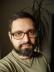## The Smallest Non-Prime Euclid Number

An Euclid number is a number that equals 1 + product of a sequence of primes.

To speed things up, I used an object that caches the sequence of primes discovered so far. The method `size` returns the length of the sequence of primes; `extend_to` extends the sequence up to the specified number.

``````package My::Primes;

sub new { bless , shift }

sub is_prime {
my (\$self, \$n) = @_;

\$self->extend_to(\$n) if \$n > \$self->[-1];
return grep \$_ == \$n, @\$self
}

sub extend_to {
my (\$self, \$n) = @_;
for my \$p (\$self->[-1] + 1 .. \$n) {
my (\$i, \$is) = (0, 1);
while (\$self->[\$i] <= sqrt \$p) {
\$is = 0, last if 0 == \$p % \$self->[\$i++];
}
push @\$self, \$p if \$is;
}
}

sub size { scalar @{ \$_ } }``````

The correctness can be verified by a simple test suite:

``````#!/usr/bin/perl
use Test::More;
use My::Primes;

my \$p = 'My::Primes'->new;

ok \$p->is_prime(2);
ok \$p->is_prime(101);

\$p->extend_to(2060);
is \$p->[-1], 2053;

done_testing();``````

Finding the first non-prime Euclid number is now easy:

``````#!/usr/bin/perl
use warnings;
use strict;
use feature qw{ say };

use List::Util qw{ product };

my \$p = 'My::Primes'->new;
my \$size = 1;
my \$e = 3;
while (\$p->is_prime(\$e)) {
++\$size;
\$e = 1 + product(@\$p[0 .. \$size - 1]);
}

say \$e;
``````

I feared the `is_prime` method would be ineffective, as it uses grep over the whole sequence. Therefore, I tried to implement a faster one using binary search:

``````sub is_prime {
my (\$self, \$n) = @_;

if (\$n > \$self->[-1]) {
\$self->extend_to(\$n);
return \$self->[-1] == \$n

} else {
my (\$from, \$to) = (0, \$#\$self);
while (\$from != \$to) {
my \$between = int((\$from + \$to) / 2);
if (\$n > \$self->[\$between]) {
\$from = \$between + 1;
} else {
\$to = \$between;
}
}
return \$self->[\$from] == \$n
}
}``````

Surprisingly, there’s no measurable difference in speed of the two implementations.

## Common Path

The basic observation that greatly simplifies the task: you don’t need to compare each path to all others. As the common path is common to all of them, you can just take one of them and compare it to all the remaining ones.

We start with the minimum set to the length of the first path. We than compare it to each remaining path, finding the first index where they start to differ. The minimum of these indices is the length of the common path.

When comparing two arrays, we need to stop the loop when the shorter array is exhausted. I used the ternary operator to find the shorter array, but using List::Util`::min` would work, as well.

``````#!/usr/bin/perl
use warnings;
use strict;
use feature qw{ say };

use List::Util qw{ first };

my @paths = qw(
/a/b/c/d
/a/b/cd
/a/b/cc
/a/b/c/d/e
);

my @p1 = split m{/}, \$paths;
my \$min = @p1;
for my \$i (1 .. \$#paths) {
my @p2 = split m{/}, \$paths[\$i];
my \$max = (@p1 < @p2) ? \$#p1 : \$#p2;
my \$diff = first { \$p1[\$_] ne \$p2[\$_] } 0 .. \$max;
\$diff //= \$max + 1;
\$min = \$diff if \$diff < \$min;
}

say join '/', (split m{/}, \$paths)[0 .. \$min - 1];``````

To test the edge cases, try running your solution against a group of equal paths, or paths that are prefixes of the longest path.I blog about Perl.